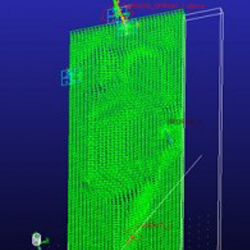Some Motivation, Why We Are Engineers:

Basic Actions of Problem Solving:

• Understanding the problem
• Simulation can be very useful to help understand what factors are important.
• Generate possible solutions to the problem
• The solutions that the designer can come up with is very dependent on the designer experience and knowledge.
• Evaluate solutions
• Simulation can provide very valuable insights during design evaluation.
• Decide which solution is best.
• Designer must perform comparisons between problem formulation and design evaluation results.

Inverse Kinematics Using Adams: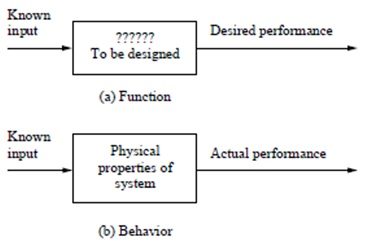• Digital reverse engineering
• Drive 0 DOF mechanisms and measure the forces required to cause motion.

• Design Evaluation
• Replace ideal motions and find the actual performance of mechanism.

Design Updates to Door: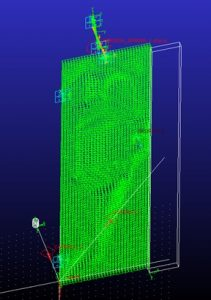• Damper mechanism

• Make rigid door flexible
• Contact between wall and door
• Contact between door and door stopper
• Ideal door opening motion
• Parameterise door model

• Flexible component is the focus
• Question: What is the system doing to my component?
• Improved model fidelity
• Model performance is the focus
• Question: What is the flexible component doing to my system?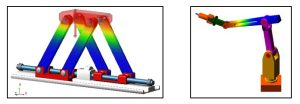Forces in Adams: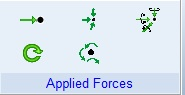•  Single Component Force
• Runtime direction
• Action only
• Single Component Torque
• Runtime direction
• action only
• Torque vector
• Force Vector
• General Force

• Ideal rigid body contact:
• Infinitely stiff
• No damping
• Impact contact uses a penalty method to approximate ideal rigid body contact.
• Nonlinear stiffness
• Linear damping with displacement based smoothing function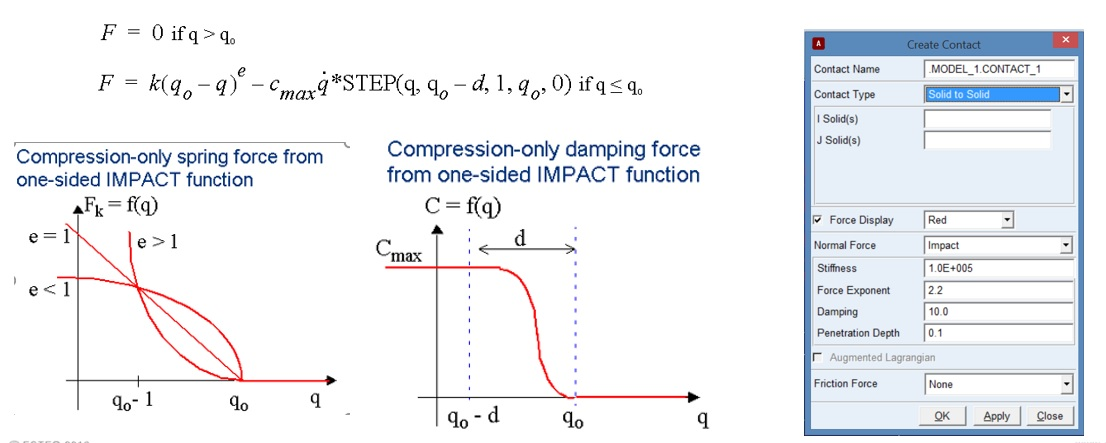• Use Hertz contact to approximate contact stiffness.
• For damping, ensure that contact bodies penetrate sufficiently, to reach specified Cmax.

Function Builder Overview:

Function builder is used to help the user define mathematical functions in Adams.

There are two function builder modes, and the functions the user has access to depends on the current mode.

• Expression mode: Used for parameterisation and design study functions.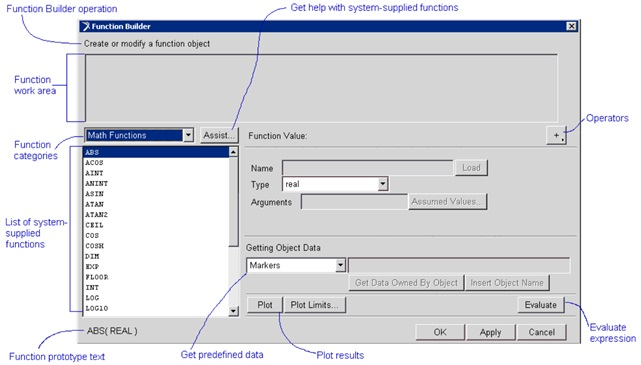• Run time mode: Used to define functions that are evaluated during a simulation.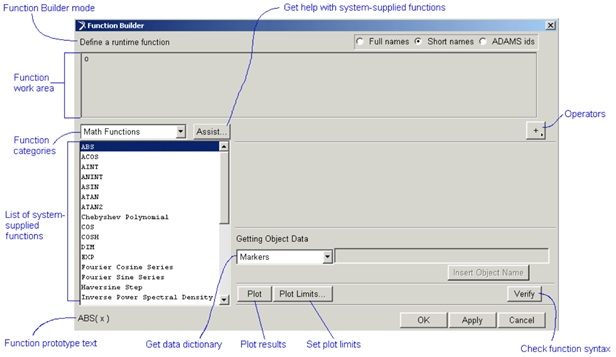• To access the function builder:
• In run time mode
• Right click and select function builder
• Use '...' button
• In expression mode
• Right click -> parameterise -> expression builder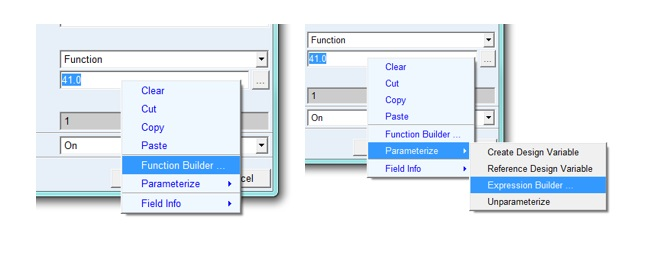• Important information:
• Adams is not case sensitive on windows
• To specify powers use '**' (e.g. 2^2 = 2**2 in Adams)
• Simulation time = time
• Pi = pi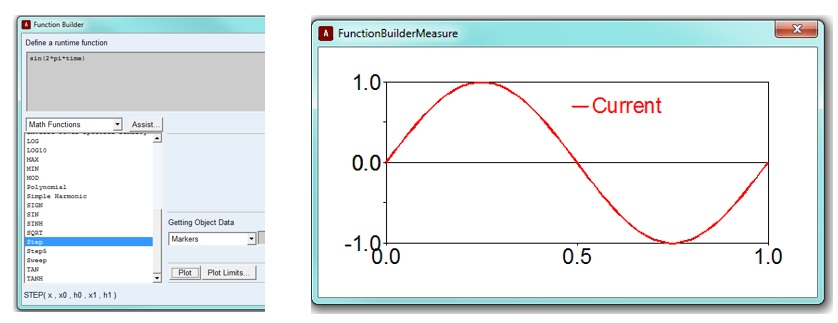Design Variables: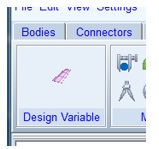• Define independent parameters that can be tied to objects
• Used during DOE's and optimisation studies

Step Function:

• Aproximates an ideal mathematical step
• Used to switch quantities on or off
• Syntax:
• Step( x , x1 , y1 , x2 , y2 )
• x - independent variable
• x1 - initial value of independent variable
• f1 - initial step function value
• x2 - final value
• f2 - final step function value
• Note: x1 < x2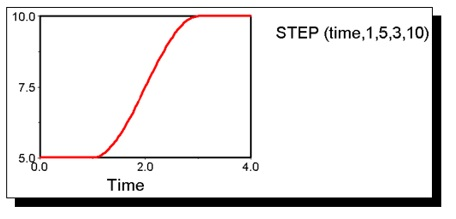Spline Functions:

• The model may require the use of catalogue or test data:
• Empirical data from suppliers:
• Nonlinear compliances
• Curves for torque v.s. motor speed
• Measured data:
• accelerometer data
• tyre lateral force a a function of lateral slip

This data can be incorporated using a spline and one of the two interpolation functions:

• Cubic-fitting method: CUBSPL
• Akima-fitting mehod: AKISPL• The Akima spline function:
• Syntax: AKISPL(x, z, spline,iord)
• x - Independent variable
• z - Optional second independent variable (set to 0 if not used)
• Spline - Name of spline containing required characteristic
• iord - order of the interpolated point (usually = 0)

Displacement Functions: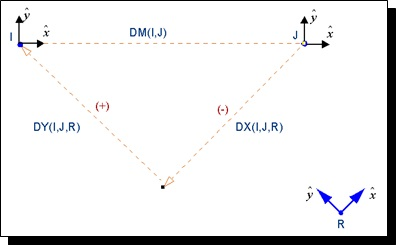Sensors and Simulation Scripts:

Deactivating a joint based on its reaction force, by using a sensor and a simulation script.

Design Studies:

Trial and error method:Design Study: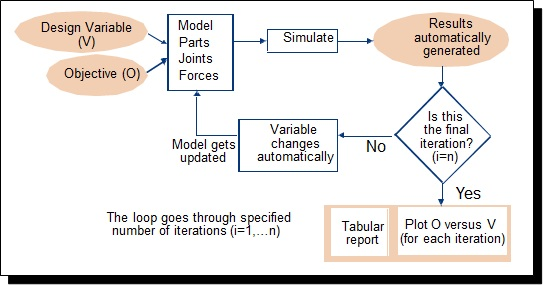Back to Lecture Index